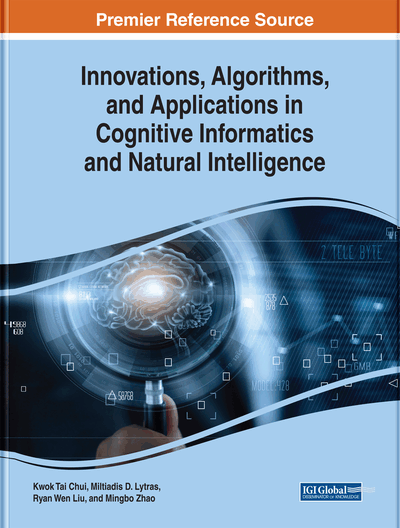# Development of the Enhanced Piece-Wise Linear Neural Network Algorithm

Veronica K. Chan (Faculty of Engineering and Applied Science, University of Regina, Canada) and Christine W. Chan (Faculty of Engineering and Applied Science, University of Regina, Canada)
DOI: 10.4018/978-1-7998-3038-2.ch006
Available
\$37.50
No Current Special Offers

## Abstract

This chapter discusses development, application, and enhancement of a decomposition neural network rule extraction algorithm for nonlinear regression problems. The dual objectives of developing the algorithms are (1) to generate good predictive models comparable in performance to the original artificial neural network (ANN) models and (2) to “open up” the black box of a neural network model and provide explicit information in the form of rules that are expressed as linear equations. The enhanced PWL-ANN algorithm improves upon the PWL-ANN algorithm because it can locate more than two breakpoints and better approximate the hidden sigmoid activation functions of the ANN. Comparison of the results produced by the two versions of the PWL-ANN algorithm showed that the enhanced PWL-ANN models provide higher predictive accuracies and improved fidelities compared to the originally trained ANN models than the PWL-ANN models.
Chapter Preview
Top

## Introduction

Artificial neural network (ANN) is a popular data mining approach because of its high classification or predictive accuracy, and resistance to noise. However, in addition to high prediction accuracy, researchers often need to gain better understanding of the problems at hand, and ANNs are black boxes that cannot be interpreted. To overcome this deficiency, the rule extraction approach can be adopted so as to generate explicit information from the analysis results of the trained ANNs. The objective of this study is to develop a decompositional neural network rule extraction algorithm for non-linear regression problems. The approach adopted is to model a given dataset using the ANN approach and the originally trained model is assumed to be a three-layer feed-forward backpropagation neural network with a sigmoid activation function. Since these are the most common types of neural network models, this will be the target models on which rule extraction will be performed. Also, based on experience, one hidden layer in an ANN is typically sufficient to solve most non-linear problems without overfitting. Although the pedagogical type of rule extraction algorithms are better in terms of computational complexity and generality than decompositional algorithms, the decompositional approach is the focus because the objective is to explore the trained neural network and “open the black box”. The algorithm approximates the activation functions of a given ANN model with piece-wise linear (PWL) equations and generates explicit information in the form of numerical formulae. The targeted problems are regression problems related to engineering domains. In terms of expressive power, we aim to generate “rules” expressed as linear numeric functions in the form of

Y = a1x1 + a2x2+… + b.

Since one of the research objectives is to understand the working mechanisms of the trained neural network model, fidelity to the trained ANN will be the primary evaluation criterion of the developed algorithm.

This paper is organized as follows: Section 2 discusses some background literature related to the area of ANN rule extraction algorithms and applications of artificial neural network modeling to engineering domains. Section 3 discussed the methodology of the PWL-ANN algorithm. Section 4 discusses some results from application of the PWL-ANN algorithm and their analysis. Section 5 presents the methodology of the Enhanced PWL-ANN algorithm as well as some application results and their analysis. Section 6 describes a comparison of the performances of the two versions of the PWL-ANN algorithms in terms of predictive accuracy and fidelity. Section 7 gives the conclusion and some directions for future work.

## Complete Chapter List

Search this Book:
Reset